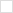TWiki>P1076 Web>Vhdl2019CollectedRequirements>FixedFloatUpdates (2020-02-17, JimLewis)

# Bug fixes and consistency updates to numeric_std, fixed_generic_pkg, and float_generic_pkg.

## Numeric_Std

### Bugzilla 15:: Extend Resize

Should numeric_bit/std have resize with size_res parameter? David proposed it for consistency with the fixed and floating point packages

### Bugzilla 17:: add_carry for unsigned/signed

In the fixed point package, but not floating point package. Not in numeric_std. Add for consistency?

Add overloads for functions to_unsigned(x) and to_signed(x) to support usage as defined by proposal Function Knows Return Vector Size.

## Fixed_generic_pkg

procedure add_carry ( L, R : in UNRESOLVED_ufixed; c_in : in STD_ULOGIC; result : out UNRESOLVED_ufixed; c_out : out STD_ULOGIC)

procedure add_carry ( L, R : in UNRESOLVED_sfixed; c_in : in STD_ULOGIC; result : out UNRESOLVED_sfixed; c_out : out STD_ULOGIC)

in each of these modify line assigning "c_out" to be as follows: c_out := result_slv(left_index-right_index);

### Fixed point documentation: Fixed the size returned by the signed reciprocal in Table xxx

Bugzilla 284Appendix G.4.3 gives the sizing rules for fixed point numbers. The rules for Signed reciprocal and unsigned reciprocal are reversed.

### Bugzilla 262:: Range Checking

Fixed Generic Pkg and checking valid range

### Conversion error in to_sulv

The parameter arg is directly converted to STD_ULOGIC_VECTOR, but this is not correct as there are negative indexes for arg which aren't in the range of the index type of std_ulogic_vector. Better to use a target type with defined index ranges, like that:

```  subtype result_subtype is STD_ULOGIC_VECTOR (arg'length-1 downto 0);
variable result : result_subtype;
...
result := result_subtype (arg);```

### Overflow in remainder

The bounds of lresize are correctly protected against overflow, but those of rresize aren't. The issue is that r'low can be integer'first, so r'low - guard_bits may overflow.

### Procedure skip_whitespace assumes l'left = 1

The procedure skip_whitespace incorrectly assumes that left bound of the string accessed by L is 1. Better to use more portable code:

```      c := l (l'left);
if (c = ' ' or c = NBSP or c = HT) then```

## Float_generic_pkg

### Modify to_float for unsigned/signed:

```function to_float ( arg : UNSIGNED;
constant exponent_width : NATURAL := float_exponent_width; -- length of FP output exponent
constant fraction_width : NATURAL := float_fraction_width; -- length of FP output fraction
constant round_style : round_type := float_round_style) -- rounding option
return UNRESOLVED_float

function to_float ( arg : SIGNED;
constant exponent_width : NATURAL := float_exponent_width; -- length of FP output exponent
constant fraction_width : NATURAL := float_fraction_width; -- length of FP output fraction
constant round_style : round_type := float_round_style) -- rounding option
return UNRESOLVED_float```

Mofify the "arg" argument in both functions to be "UNRESOLVED_UNSIGNED" and "UNRESOLVED_SIGN". The type of the "XARG" variable must be modified accordingly.

### Modify to_float for sfixed:

```function to_float ( arg : UNRESOLVED_sfixed; -- signed fixed point constant
exponent_width : NATURAL := float_exponent_width; -- length of FP output exponent constant
fraction_width : NATURAL := float_fraction_width; -- length of FP output fraction constant
round_style : round_type := float_round_style; -- rounding constant
denormalize : BOOLEAN := float_denormalize) -- rounding option
return UNRESOLVED_float```

Modify the variable "arg_int" to be as follows:

`variable arg_int : UNSIGNED(integer_width - in_fraction_width downto 0); -- unsigned version of argument `

Modify the variables "exp" and "exptmp" as follows:

`variable exp, exptmp : SIGNED (exponent_width + 1 downto 0);`

Modify the assignment of "arg_int" on line 2924 from "argx := -argx;" to:

`arg_int := UNSIGNED(to_x01(not STD_LOGIC_VECTOR (argx))) + 1; -- Make it positive with two's complement `

Modify the assignment of "arg_int" on line 2927 to the following:

`arg_int := UNSIGNED(to_x01(STD_LOGIC_VECTOR (argx))); -- new line: direct conversion to unsigned`

### Conversion error in to_sulv

Same issue as in the fixed_generic_pkg package.

### Procedure skip_whitespace assumes l'left = 1

Same issue as in the fixed_generic_pkg_package.

Add overloads for functions to_unsigned(x) and to_signed(x) to support usage as defined by proposal Function Knows Return Vector Size.